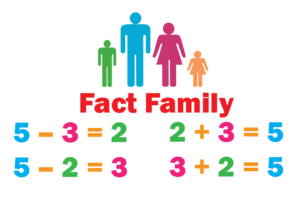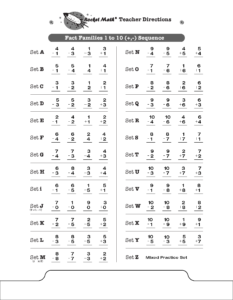## Add-Subtract Fact Families to 10 Learning Track

A number of math programs around the country introduce math facts in families.  Now Rocket Math does too!  A fact family includes both addition and subtraction facts. You can see below the 25 examples of fact families taught in this program starting with Set A; 3+1, 1+3, 4-1 & 4-3.  The sheet shows the sequence of learning facts in the new Rocket Math  program Add-Subtract Fact Families to 10.  Each set that students learn from A to Y adds just one fact family to be learned, so it isn’t too hard to remember.  (That’s the Rocket Math secret ingredient!)Learning math facts in families, is gaining in popularity these days.  Logic suggests that this would be an easier way to learn.  However, the research is not definitive that this is easier or a faster way to learn facts than separating the operations and learning all addition facts first and then learning all subtraction facts.  But learning in fact families is a viable option, and we wanted to have it available for Rocket Math customers.

Flash news!! Someone looking for a master’s or doctoral thesis could do a comparative study of students using the fact families vs. the separated facts in Rocket Math. This could easily be a gold standard research study because you could randomly assign students to conditions within classrooms–the routine is the same for both–just the materials in their hands is different!  Just sayin’…

Best fit for first grade.  Dr. Don separated out the facts to 10 from the 11s-18s, because these 25 families are just enough for one Rocket Math program.  It is a good and sufficient accomplishment for first grade.  Some first grades prefer to keep the numbers small but to learn both addition and subtraction–so this program accomplishes that.

Add-Subtract Fact Families to 10 is the first half.  The rest of the addition and subtraction fact families, which students could learn in 2nd grade, are the Add-Subtract Fact Families 11 to 18.  We most sincerely want students to be successful and to enjoy (as much as possible) the necessary chore of learning math facts to automaticity.